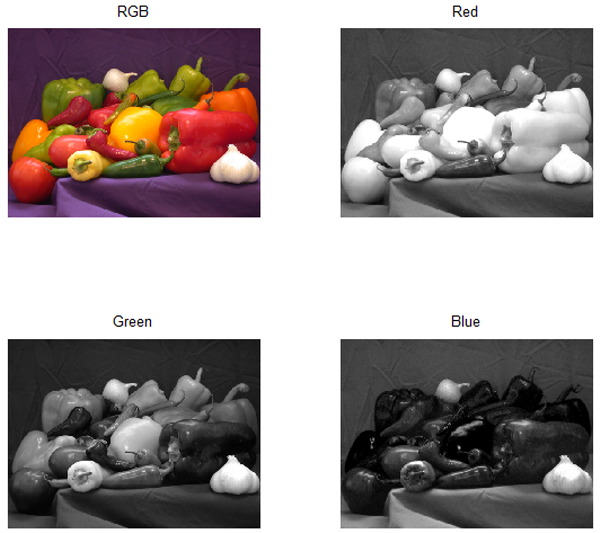# When Deal doesn’t work, Disperse!

Brett's Pick this week is Disperse, by Sam Hallman.

When I first saw Sam's "disperse" function--with the tagline "Assign elements of an input array to individual output variables with a single function call--" I thought, "well, that's silly! Why not just use MATLAB's deal function?" Deal lives to distribute inputs to outputs.

But fortunately for me, I read on, and came to Sam's statement: "DISPERSE is not the same as DEAL. For example, if A is a vector then [a b c d] = disperse(A) performs a=A(1), b=A(2), c=A(3), and d=A(4), whereas [a b c d] = deal(A) performs a=A, b=A, c=A, and d=A." That immediately had me thinking about all the many times over the years when I've looked to DEAL to do something it wasn't designed to do, and ended up writing for-loops, or clumsy work-around code, instead. But no longer!

Sam's code is terse, yet widely useful. It works beautifully on cell arrays:

data = {'one' [2 3] 'four' 5}
[a,b,c,d] = disperse(data)
data =
Columns 1 through 3
'one'    [1x2 double]    'four'
Column 4

a =
one
b =
2     3
c =
four
d =
5


Compare that to:

[a,b,c,d] = deal(data)
a =
Columns 1 through 3
'one'    [1x2 double]    'four'
Column 4

b =
Columns 1 through 3
'one'    [1x2 double]    'four'
Column 4

c =
Columns 1 through 3
'one'    [1x2 double]    'four'
Column 4

d =
Columns 1 through 3
'one'    [1x2 double]    'four'
Column 4



DISPERSE works with the elements of structs, too, where a usage of DEAL would similarly assign the full structure to each of the outputs:

s = struct('strings', {'sam','hello'}, 'lengths', [3 5])
[a b] = disperse(s)
s =
1x2 struct array with fields:
strings
lengths
a =
strings: 'sam'
lengths: [3 5]
b =
strings: 'hello'
lengths: [3 5]


Note that we could workaround the cell problem with this approach:

[a,b,c,d] = deal(data{:})
a =
one
b =
2     3
c =
four
d =
5


And for structures, we could write something like this:

sc = num2cell(s);
[a, b] = deal(sc{:})
a =
strings: 'sam'
lengths: [3 5]
b =
strings: 'hello'
lengths: [3 5]


But the simplified syntax and ease of use still recommends DISPERSE.

You can subset your data, extract (disperse) elements of an n-dimensional array, even extract individual color planes in an RGB image:

img = imread('peppers.png');
[r g b] = disperse(img);
% figure;
% subplot(2,2,1)
% imshow(img); title('RGB')
% subplot(2,2,2)
% imshow(r); title('Red')
% subplot(2,2,3)
% imshow(g); title('Green')
% subplot(2,2,4)
% imshow(b); title('Blue')DISPERSE ships with an html report, generated with MATLAB's PUBLISH function, that shows lots of ways to use the function. It's thorough, and very helpful.